# Lyndon word

(diff) ← Older revision | Latest revision (diff) | Newer revision → (diff)

A word is a sequence of letters, that is, elements chosen from a setcalled an alphabet. A word is usually written as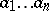, or abbreviated by a single symbol: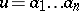. The length of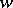is equal to the number of letters in, i.e.. One may concatenate two words,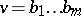, and this operation is concisely written as. Words may be ordered lexicographically; more precisely, given a total order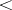on, one may extend it over the set of words by settingif either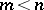andor there exists ansuch thatwith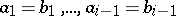and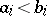. This word order is sometimes referred to as alphabetical order. For more details, see [a1].

Lyndon words are words strictly less than any of their non-empty proper right factors (or strict suffixes), that is,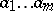is a Lyndon word if it is strictly less thanfor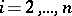(with respect to the lexicographic order). These were introduced by R.C. Lyndon [a2] for constructing bases of the lower central series for free groups (cf. Central series of a group). From the definition one can see that letters are Lyndon words. Equivalently,is a Lyndon word if it is strictly less than any of the circular shifts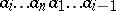(). One important characterization of these words yields a recursive process to generate them: a wordis a Lyndon word if and only if there exists two Lyndon words,such thatand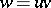. J.P. Duval [a3] gave a beautiful, and clever, algorithm that generates Lyndon words of bounded length over a finite alphabet. The number of Lyndon words of lengthover a finite alphabet of cardinalitymay be explicitly computed, using Witt's formula: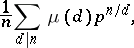whereis the Möbius function.

A multi-homogeneous version of Witt's formula may be found in [a4]. The central result about Lyndon words is the following Chen–Fox–Lyndon theorem: Any word can be expressed as a unique non-increasing product of Lyndon words. The preceding characterization suggests an algorithm to compute this factorization. Duval [a5] gave a simple and efficient algorithm that factorizes a word in linear time.

The applications of Lyndon words, in algebra and combinatorics, seem innumerable. For instance, there are as many Lyndon words over an alphabet of cardinality(a prime) as there are irreducible polynomials over a finite field of characteristic; see [a4]. The factorization theorem, when applied to permutations (considered as words without repetitions), yields a variant of the so-called Foata transform [a1], Chap. 10.

Given any Lyndon word, one may compute the factorization ofas a product, whereis a Lyndon word of maximal length (less than the length of). The strict suffix (or proper right factor)can also be characterized as the smallest strict suffix of. Thenis also a Lyndon word and. This factorization ofis called its right standard factorization. Similarly, one may define its left standard factorization. Letbe a mapping from the set of Lyndon words into the free associative algebra onby setting, and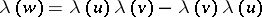, whereis its right standard factorization. The polynomial thus associated tois a Lie polynomial, and the setforms a linear basis of the free Lie algebra over(see Lie algebra, free). Note that the iterated right standard factorizations recursively define a bracketing of(see Binary tree), and thus define a Hall set. The corresponding basis is called the Chen–Fox–Lyndon basis, or simply the Lyndon basis. It is practically identical with the Shirshov basis.

A good introduction to Lyndon words is [a1], Chap. 5. For applications of Lyndon words related to free Lie algebras, see [a4].